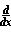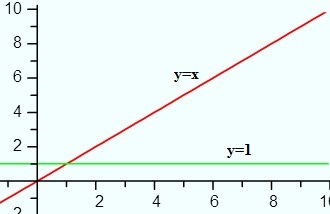# Common Derivatives

## The derivative of sin3x

The derivative of sin3x is 3sin2x cos x.
There are two main ways to arrive at the derivative, either by using the definition of a limit (the long way), or by using a shortcut, called the general power rule. Shortcuts exist so that you can skip using the long way of finding a derivative: the definition of a limit. The general form of the power rule helps you to differentiate functions of the form [u(x)]n], like sin3x, which can be rewritten as [sin x]3, which has the inside function of “sin x” and the outside function of x3. The general form of the power rule is:
If y-un, then y = nun – 1*u’, where “u” is the inside function.

Example problem: Find the Derivative of Sin3x

Step 1: Rewrite the equation to make it a power function:
sin3x = [sin x]3

Step 2: Find the derivative for the “inside” part of the function, sin x. According to the general rules for differentiation, the derivative of sin x is cos x:
f’ sin x = cos x

Step 3: Rewrite the function according to the general power rule. In other words, write out the general power rule, substituting in your function where appropriate. The last half of the general power rule is the derivative of the inside function you worked out in Step 2:
f- = 3[sin x]3-1[cos x] = 3[sin x]2[cos x]

Step 4: Rewrite using algebra:
3[sin x]2[cos x] = 3sin2x cos x

That’s it!

Tip: Calculus uses a lot of algebra and trigonometry. If your algebra skills are weak, this is where the course will likely become difficult. Rather than concentrating on memorizing the rules of differentiation, concentrate on improving your algebra skills. Being able to look at a function and seeing which rule might apply if you manipulate the equation (for example, knowing that a square root can be rewritten as “to the 1/2 power”) is key to working out derivatives.

## Common Derivative Rules

This is a list of the more common derivatives (the ones you’ll usually find in the appendix of a text book).
Power of xc = 0x = 1xn = n x(n-1)

Exponential / Logarithmic Derivatives Tableex = exbx = bx ln(b)ln(x) = 1/x

Trigonometricsin x = cos xcsc x = -csc x cot xcos x = – sin xsec x = sec x tan xtan x = sec2 xcot x = – csc2 x

Inverse Trigonometricarcsin x = 1 / (√ (1- x2))arccsc x = -1 / (|x| √ (x2 – 1))arccos x = -1 / (√ (1- x2))arcsec x = 1 / (|x| √ (x2) – 1)arctan x = 1 / (1 + x2)arccot x = (-1 / 1 +x2)

Hyperbolic Functionssinh x = cosh xcsch x = – coth x csch xcosh x = sinh xsech x = – tanh x sech xtanh x = 1 – tanh2xcoth x = 1 – coth2x

Above is a list of the most common derivatives you’ll find in a derivatives table. If you aren’t finding the derivative you need here, it’s possible that the derivative you are looking for isn’t a generic derivative (i.e. you actually have to figure out the derivative from scratch). If that’s the case and you need to find the derivative, search this site, or try an online calculator like this one from Wolfram Alpha.

## Common Derivatives: x

Watch the video, or read on below:

The derivative of x = 1. Similarly, the derivative of -x = 1.

## Why is the Derivative of x equal to just 1 ?

The definition of a derivative is the slope of the tangent line at any point on the graph. The function y = x is a constant function. It has a positive slope of exactly 1 at all points on the graph—that’s why the derivative for the whole function is defined as 1.The graph of -x shows it is a decreasing function with a negative slope of exactly -1 at all points:Graph of y=-x (red line) and the derivative, -1 (green line).

One you wrap the idea around your head that the derivative is just the slope of the tangent line, it makes finding many common derivatives extremely easy. If only all derivatives in calculus were this simple!

## What About Other Functions with Constants?

The derivative of any other functions with some value of x, times a constant, is just the constant. For example:

• The derivative of 12x is 12,
• The derivative of 10,000x is 10,000.

You can apply this rule to any x value multiplied by a constant, including π (see pi derivative), e (Euler’s number), decimal numbers, fractions and other constants.

## Common Derivatives: 2x

Watch the video or read on below:

## Formula

The derivative of any x value multiplied by a constant is just the constant itself.
As a formula, that’s: [cx]′ = c
In words, that’s saying if you have an x-value multiplied by any constant, the derivative (denoted by the ′ symbol, which is called “prime notation“) is just the constant.

For example, the derivative of 2x is 2, or the derivative of 100x is 100. You can apply this rule to any x value multiplied by a constant, including:

• pi,
• e,
• decimals,
• fractions.

The rule can easily be extended to find the derivative of 3x (which is 3), the derivative of 4x (which is 4) …∞. Just drop the “x”, and you have the derivative.

## Why is the Derivative of 2x just “2”?

The derivative is a tangent line at a point. In other words, find the slope at a point and you have the derivative. The slope of the line 2x is 2, no matter what point you pick to find the slope. Therefore, the derivative of the entire function is 2.Graph of y = 2x (red line) and the derivative of 2x(green line).

Tip: Just in case you need a refresher, the slope formula is: change in y / change in x. You can use this formula to take an average of a slope at two points; as the slope for a linear graph (like 2x) is constant, finding the slope between two points will also give you the derivative of 2x.

## Common Derivatives: Derivative of 3x

Watch the video, or read on below:

The derivative of 3x is 3.

The derivative of any x multiplied by a constant is just the constant. For example:

• The derivative of 99x is 99,
• The derivative of 101x is 101.

## Why is the derivative of 3x just 3?

The derivative is the tangent line at a point. In other words, for straight line functions like this, all you do is find the slope at a particular point and that value is the derivative.

You can find the slope of a line between two points by using the slope formula:

Slope = Change in y / change in x.

As you can probably deduce from the formula, it’s impossible to find the slope at a point…because there’s no change! In calculus, if you want to find the slope at a point you just pick a couple of points that are very close to the point you want to find the slope for. How close is close enough? That’s usually a matter of opinion, but as long as you’re “in the area” (in other words, as long as you’re reasonably close), you should be “close enough.”

For example, if you want to find the derivative of 3x (which is just the slope), you might pick the point x = 3 to find the derivative at. To use the slope formula, you need two points, so you could choose x = 2 and x = 4 (which are 1 units either side of 3). A linear function has a constant slope — so it actually doesn’t matter which points you pick: the function has the same constant derivative.

The slope of the line 3x is 3, no matter what points you pick to find the slope. Therefore, the derivative of 3x at point x = 2 to x = 4 is the derivative of the entire function.

## Common Derivatives: TI 89/Titanium Steps

Example problem: Find the derivative of f(x) = 3x on the TI 89.

Step 1: Press The F3 button.

Step 2: Select “1: d( differentiate”. You can either use the down arrow key or type the number to to select it.

Step 3: Press ENTER. The calculator will fill in the command line on the home screen with d(

Step 4: Type your function name, followed by a comma. For example, if your function is 3x then type “3x,”. The syntax will now look like this:Step 5: Type X.This tells the calculator you’re differentiating with respect to X.

Step 6: Type a closing parenthesis symbol.Step 7: Press ENTER. The solution is shown on the right hand side of the screen.The derivative of 3x is 3.

Warning: the letter d for derivative is not the same as the letter D on your keyboard. In other words, you can’t just type in “d(” into the home screen. You have to hit the F3 key (you could also search for it in the catalog, but why do it the long way?).

## Common Derivatives: e, and ex

Contents:

1. Derivative of e
2. Derivative of ex

## Derivative of e

The derivative of e is 0.

Why?

Because the derivative of any constant function is 0.

Euler’s number (e), sometimes called Napier’s constant, is not a variable like x or y. It’s a constant like π. It has a value of approximately 2.718.

This graph shows the constant function y = e (red) and y = ex (green):If you look at the graph of e, you can see that the slope is zero for all points on the line; A horizontal line always has a slope of zero. Therefore, the derivative is always zero for constant functions (like e) that produce a horizontal line when graphed.

## Derivative of ex

The derivative of ex is ex.

Why?
This is an unusual function because it is its own derivative. In other words, the slope is the same as the function output (the y-value) for all points on the graph. To make this clearer, try graphing the function and finding the slope at certain points.The above image shows that the derivative (i.e. the slope of the tangent line) at point (0, 1) is 1. Let’s imagine you were starting to graph your derivative. For that one single point on your function (0, 1), the first point on your derivative function would lie on the line of the function y = 1. That point is shown on the red line below:

So far, we only have a single point on our derivative. We need a few more to start graphing the derivative function. Next, let’s look at the slope for x = 1:## Derivative of e functions Using the Chain Rule

The derivative of ex is actually a special case of a slightly more complicated rule called the chain rule. You use the rule when the power of e is a function of x and not just the variable x on its own.

When e when it’s combined with another function. For example, you might be asked to find the derivative of e functions that look like this: e5x or x2x2. For these functions, you need to use the chain rule.

Next: The Chain Rule.